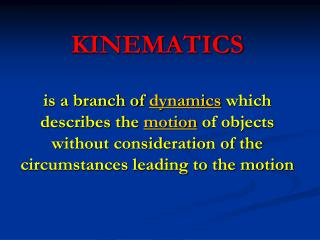DownloadDownload PresentationKINEMATICS

# KINEMATICS

Download Presentation## KINEMATICS

- - - - - - - - - - - - - - - - - - - - - - - - - - - E N D - - - - - - - - - - - - - - - - - - - - - - - - - - -
##### Presentation Transcript

1. KINEMATICS is a branch of dynamics which describes the motion of objects without consideration of the circumstances leading to the motion

2. FRAME OF REFERENCE • a set of axes relative to which an observer can measure the position and motion of all points in a system, as well as the orientation of objects in it. • Coordinate axes are used to represent the frame of reference

3. Moving or Not Moving? Click to play Reference Frame Video

4. Motion depends on perspective

5. SCALAR QUANTITY • A quantity define by magnitude only (Ex) 5 meters 4 kg 50 kilometers per hour (kph) 60 degrees time, distance, speed, temperature, mass, energy

6. VECTOR QUANTITY A quantity that requires both magnitude and direction. (Ex) 5 meters north (5 m N) 35 km NNE 65 newtons to the left (65 N left) 13 m/s down velocity, displacement, force, momentum, electric and magnetic fields

7. DIRECTIONS 22.5 o W of N 67.5 o N of W 0 90 270 180

8. Vectors are usually named with capital letters with arrows above the letter. They are represented graphically as arrows. The length of the arrow corresponds to the magnitude of the vector. The direction the arrow points is the vector direction. Examples : A = 20 m/s at 35° N of E B = 120 m at 60° S of E C = 5.8 m west

9. VECTOR ADDITION GRAPHICAL METHOD • The vectors are added using the head-to-tail method. Magnitude can be measured and the direction determined through the use of a protractor (Ex.) = =

10. TRIGONOMETRIC METHOD 5 4 Roman Road 3 The secret of how Roman engineers were able to construct “straight as an arrow” roads is a piece of rope with 11 knots folded into three sections with the following ratio: 3:4:5.

11. SOH CAH TOA

12. Resolving a Vector Into Components +y The horizontal, or x-component, of A is found by Ax = A cos q. A Ay q Ax +x The vertical, or y-component, of A is found by Ay = A sin q. By the Pythagorean Theorem, Ax2 + Ay2 = A2. Every vector can be resolved using these formulas, such that A is the magnitude of A, and q is the angle the vector makes with the x-axis. Each component must have the proper “sign” according to the quadrant the vector terminates in.

13. Analytical Method of Vector Addition 1.Find the x- and y-components of each vector. Ax = A cos q = Ay = A sin q = By = B sin q = Bx = B cos q = Cx = C cos q = Cy = C sin q = Rx = Ry= 2. Add the x-components. This is the x-component (Rx) of the resultant. 3. Add the y-components. This is the y-component (Ry) of the resultant. 4. Use the Pythagorean Theorem to find the magnitude of the resultant vector. Rx2 + Ry2 = R2

14. 5. Find the reference angle by taking the inverse tangent of the absolute value of the y-component divided by the x-component. q = Tan-1Ry/Rx 6. Use the “signs” of Rx and Ry to determine the quadrant. NW NE (-,+) (+,+) (-,-) (+,-) SW SE

15. Θ Θ = tan-1 opp = tan -1 11 = 45 o adj 11

16. EFFECT OF WIND ON A PLANE’S VELOCITY

17. RESULTANT VELOCITY OF A CROSSWIND ON A PLANE’S VELOCITY

18. EFFECT OF RIVER CURRENT ON A RIVERBOAT’S MOTION